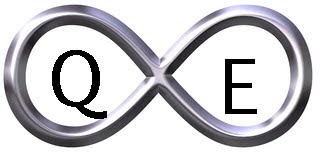### AmenRa's Corner 1/29/16

FOMC Meeting…Creditcane™: REPETERE AD INFINITUM: CAVEAT EMPTOR.

SPY (SPDR S&P 500)
-change (above mid)
trend=down
low= 187.81
rev= 197.63; mid= 192.72

monthly info -no change (above mid)
trend=no; direction=down (2 bars)
low= 191.63; rev= 211.14; mid= 201.39

XLB (SPDR Materials)
-no change (below mid)
trend=down
low= 38.25
rev= 43.94; mid= 41.10

monthly info new low 38.77
trend=down
low= 38.77; rev= 45.95; mid= 42.36

XLE (SPDR Energy)
-change (above mid)
trend=down
low= 54.35
rev= 60.46; mid= 57.41

monthly info new low 58.21
trend=down
low= 58.21; rev= 66.43; mid= 62.32

XLF (SPDR Financial)
-no change (below mid)
trend=down
high= 21.28
rev= 22.65; mid= 21.97

monthly info MONTHLY CONFIRMATION new low 21.72
trend=no; direction=down (2 bars)
low= 21.72; rev= 25.21; mid= 23.47

XLI (SPDR Industrials)
-no change (below mid)
trend=down
low= 48.65
rev= 52.61; mid= 50.63

monthly info -no change (below mid)
trend=no; direction=down (2 bars)
low= 49.89; rev= 57.49; mid= 53.69

XLK (SPDR Technology)
-no change (below mid)
trend=no
direction=down (1 bar)
low= 39.34
rev= 44.57; mid= 41.96

monthly info -change (below mid)
trend=no; direction=up (2 bars)
high= 43.96; rev= 39.50; mid= 41.73

XLP (SPDR Consumer Staples)
-no change (above mid)
trend=no
direction=up (2 bars)
low= 50.92
rev= 46.52; mid= 48.72

monthly info new high 50.76
trend=up
high= 50.76; rev= 50.01; mid= 50.39

XLU (SPDR Utilities)
-no change (above mid)
trend=no
direction=down (1 bar)
low= 40.96
rev= 45.57; mid= 43.27

monthly info MONTHLY REVERSAL new high 45.42
trend=no; direction=up (1 bar)
high= 45.42; rev= 41.46; mid= 43.44

XLV (SPDR Healthcare)
new low 66.47
trend=down
low= 66.47
rev= 68.68; mid= 67.58

monthly info -change (below mid)
trend=no; direction=down (2 bars)
low= 66.23; rev= 76.59; mid= 71.41

XLY (SPDR Consumer Discretionary)
-no change (below mid)
trend=no
direction=down (2 bars)
low= 71.50
rev= 81.47; mid= 76.49

monthly info MONTHLY REVERSAL new low 74.11
trend=no; direction=down (1 bar)
low= 74.11; rev= 80.97; mid= 77.54

XPH (SPDR Pharmaceuticals)
-no change (below mid)
trend=no
direction=up (1 bar)
high= 122.61
rev= 35.12; mid= 78.87

monthly info -no change (below mid)
trend=no; direction=down (1 bar)
low= 35.25; rev= 69.23; mid= 52.24

XRT (SPDR Retail)
-no change (below mid)
trend=no
direction=down (2 bars)
low= 39.41
rev= 45.56; mid= 42.49

monthly info -no change (below mid)
trend=no; direction=down (1 bar)
low= 38.94; rev= 81.68; mid= 60.31

XBI (SPDR Biotech)
new low 50.55
trend=down
low= 50.55
rev= 65.48; mid= 58.02

monthly info new low 50.55
trend=down
low 50.55; rev= 84.08; mid= 67.32

XTN (SPDR Transportation)
-no change (below mid)
trend=down
low= 37.68
rev= 43.05; mid= 40.37

monthly info new low 39.67
trend=down
low 39.67; rev= 46.03; mid= 42.85

RBOB (RBOB Gasoline)
-no change (below mid)
trend=no
direction=down (2 bars)
low= 1.04
rev= 1.37; mid= 1.21

monthly info -no change (below mid)
trend=down
low= 1.13; rev= 1.36; mid= 1.25IS IT OVER? IS IT REALLY OVER?